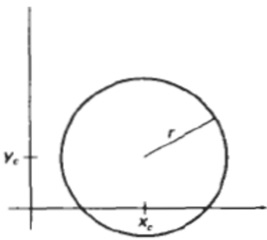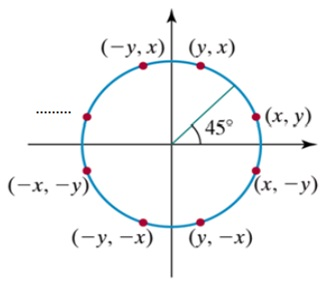# MCQ | Circle Drawing and Ellipse Drawing Algorithms in Computer Graphics

Multiple choice questions and answers (MCQ) based on the Circle Drawing and Ellipse Drawing Algorithms in computer graphics with 4 choices, correct answer and explanation.
Submitted by IncludeHelp, on April 11, 2021

Question 1: What is the equation for a circle?

1. y = mx + c
2. y2 = r2 – x2
3. rx2y2 = rx2ry2 - ry2x2
4. None of the above

Answer: b. y2 = r2 – x2

Explanation:

The Equation of circle is:

```x2 + y2 = r2
y2 = r2 – x2
```

Question 2: Which among the following is the correct equation for an ellipse?

1. y = mx + c
2. y2 = r2 – x2
3. rx2y2 = rx2ry2 - ry2x2
4. None of the above

Answer: c. rx2y2 = rx2ry2 - ry2x2

Explanation:

The Equation of an ellipse is:

```ry2x2 + rx2y2 = rx2ry2
rx2y2 = rx2ry2 - ry2x2```

Question 3: Which of the following properties is followed by the ellipse?

1. 4 – symmetry property
2. 8 – symmetry property
3. 6 – symmetry property
4. All of the above

Answer: a. 4 – symmetry property

Explanation:

An ellipse is identical through all its quadrant values. i.e., if you place the origin at the center of the ellipse, then it will have the mirror images for its points on all the four quadrants. Hence, the ellipse is said to have the 4-symmetry property.

Question 4: Which of the following are the methods to define a circle?

1. Using polynomial method.
2. Using polar coordinates methods.
3. Both a. and b.
4. None of the above

Answer: c. Both a. and b.

Explanation:

A circle can be defined from both the stated options: Using the polynomial method as well as the polar coordinates method.

Question 5: "The mid-point algorithm is used for both circle drawing as well as ellipse drawing, but the procedure is different for both of them."
Based upon the above statement, determine whether the following condition is true or false.
"The circle follows 8 - symmetry property whereas the ellipse follows 4 – symmetry property."

1. True
2. False

Explanation:

The circle is identical along all its octants whereas an ellipse is identical long all its quadrants. Hence the given statement: "The circle follows 8 - symmetry property whereas the ellipse follows 4 – symmetry property" is true.

Question 6: Why does a circle drawn on a computer screen look elliptical?

1. Because of its elliptical nature
2. Because CRTs design is elliptical
3. It is due to the ratio aspect of computer monitor
4. None of these

Answer: c. It is due to the ratio aspect of computer monitor

Explanation:

The screen aspect ratio, a circle drawn on the panel seems to be elliptical.

Question 7: With reference to properties of a circle, a circle is defined as a set of points that are all the given distance (xc ,yc).1. True
2. False

Explanation:

A circle is defined as a set of points that are all the given distance (xc ,yc).

Question 8: An ellipse consists of two axes: major and minor axes where the major axis is the longest diameter and minor axis is the shortest diameter.

1. True
2. False

Explanation:

From the image plane, an ellipse consists of two axes: major and minor axes. The major axis is the longest diameter and minor axis is the shortest diameter. Each end of the major axis is the vertex, and each end of the minor axis is the co-vertex of the ellipse.

Question 9: During a circle creation using Bresenham's algorithm, it is usually simple to generate a circle?

1. One octant first and other by successive translation
2. One octant first and other by successive reflection
3. Both A and B
4. None of the above mentioned

Answer: b. One octant first and other by successive reflection

Explanation:

Only one octant is needed in Bresehnam's algorithm, and the remaining octants can be obtained by successive reflection.

Question 10: In computer graphics, the mid-point ellipse algorithm is an ___ of drawing an ellipse.

1. Elliptical method
2. Decremented method
3. Circulation method
4. None of the above mentioned

Answer: d. None of the above mentioned

Explanation:

In computer graphics, the mid-point ellipse algorithm is an Incremental method of drawing an ellipse.

Question 11: Midpoint ellipse algorithm plots points of an ellipse on the first quadrant by dividing the quadrant into two regions.

1. True
2. False

Explanation:

A midpoint ellipse algorithm plots points of an ellipse on the first quadrant by dividing the quadrant into two regions.

Question 12: In the following figure, which one will be the appropriate pair of coordinates in the blanks?1. (-x, y)
2. (x, -y)
3. (-x, -y)
4. (x, y)

Explanation:

(-x, y) will be the correct pair of coordinates.

Question 13: The value of the decision parameter determines whether the mid-point lies ___ boundary and the then position of the mid-point help in drawing the ellipse.

1. At boundaries
2. Inside, outside, or on the ellipse
3. Only inside the boundaries
4. Only outside the boundaries

Answer: b. Inside, outside, or on the ellipse

Explanation:

The value of the decision parameter determines whether the mid-point lies Inside, outside, or on the ellipse boundary and the then position of the mid-point helps in drawing the ellipse.

Question 14: Which of the following method/methods are used to get and set the location of a pixel, object or text in a desktop's active area?

1. Gravity field method
2. Basic positioning method
3. Only A
4. None of The Above

Explanation:

In the active region of a desktop, basic positioning methods are used to get and set the location of a pixel, point, or document.

Question 15: The ___ of the circle is often used by the midpoint circle drawing algorithm to produce.

1. Two-way symmetry
2. Six-way symmetry
3. Eight-way symmetry
4. None of the mentioned above

Explanation:

The two-way symmetry is used to create a circle in the midpoint circle drawing algorithm.

Question 16: Let R be the circumference of a circle. The angle created by an arc of length R at the circle's middle is?

2. 90 degree
3. 60 degree
4. 1 degree

Explanation:

One radian is the angle generated by wrapping a circle's radius around its circumference.

Question 17: To draw a perspective image of a globe, first enclose the circle in ___ then add pointing points, and so on.

1. Circle
2. Rectangle
3. Ellipse
4. All of the mentioned above

Explanation:

The circle can be enclosed in a square to achieve points on an ellipse, and the midpoints of the sides and intersection of diagonals with the circle are 8 points. Parallel to the square's edges, lines are traced along these stages.

Question 18: ___ is defined as a group of points where the sum of the distances for all points is the same.

1. Lines
2. Dots
3. Only A
4. Ellipses

Explanation:

Ellipses are a set of lines.

Question 19: The orientation of a picture plane in relation to the source is unaffected by the perspective view's distance.

1. True
2. False

Explanation:

If an entity is set behind the picture plane, it will appear smaller in perspective. The height of the viewpoint would rise as the object is brought closer to the picture plane, and vice versa.

Question 20: At any point (x, y) on the boundary of the circle with radius r satisfies the equation fcircle (x,y)=0. If the point is in the interior of the circle, the circle function is negative while if the point is outside the circle the, circle function is ___

1. Positive
2. Also negative
3. May be positive or negative
4. None of the above mentioned

Explanation:

With reference to circle equation fcircle (x, y) = 0. If the point is in the interior of the circle, the circle function is negative while if the point is outside the circle the, circle function is positive.

More...